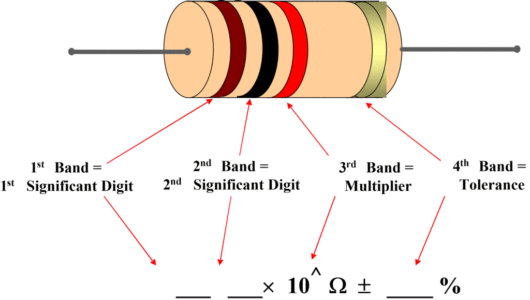Home / Basic Electrical / How to Read Resistor Color Code | Resistor Color Bands

# How to Read Resistor Color Code | Resistor Color Bands

Want create site? Find Free WordPress Themes and plugins.

Carbon resistors are color coded- that is, they have several color bands painted around the body near one end- to identify their ohmic values. Other types of resistors are not color-coded; instead, they have their ohmic values and, sometimes, identifying part numbers printed on them. The code has been established by the Electronics Industries Association (EIA) and appears in Table 1.

ColorFirst
Band
Second
Band
MultiplierTolerance
Black00$\times {{10}^{0}}$
Brown11$\times {{10}^{1}}$ $\pm 1$ %
Red22$\times {{10}^{2}}$ $\pm 2$ %
Orange33$\times {{10}^{3}}$
Yellow44$\times {{10}^{4}}$
Green55$\times {{10}^{5}}$
Blue66$\times {{10}^{6}}$
Violet77$\times {{10}^{7}}$
Grey88$\times {{10}^{8}}$
White99$\times {{10}^{9}}$
Gold--$\times 0.1$$\pm 5$ %
Silver--$\times 0.01$$\pm 10$ %
No color---$\pm 20$ %

Table.1: Standard Resistor Color Code

## Using the color code

The use of the code can be understood by referring to the resistor shown in figure 1.Fig.2: Color-Coded Carbon Composition Resistor

The first two bands of the resistor represent the first and second significant numbers and are brown and black, which, from Table 1, represent 1 and 0, respectively. The multiplier band is red, which indicates that two zeros must be added. Therefore, the resistor’s ohmic value is 1000 ohm, which frequently written as 1 kΩ (k=1000).

The tolerance band is Gold, which represents $\pm 5$%  (see Table 1). Consequently, any value between 950 and 1050 ohm is within the tolerance range and is satisfactory.

### Example

Determine the ohmic value of the carbon resistor with color bands given in the following figure.Fig.3: Color-Coded Carbon Composition Resistor for Example

### Solution

$Resistor\text{ }nominal\text{ }value~=\text{ }47*{{10}^{3}}\Omega$

$~=\text{ }47,000\text{ }\Omega =47\text{ k}\Omega$

If we consider the tolerance value then,

For minimum resistance value:

\begin{align} & =47k\Omega -47k\Omega *0.05 \\ & =47k\Omega -2.35k\Omega \\ & =44.65k\Omega \\\end{align}

For maximum resistance value:

\begin{align} & =47k\Omega +47k\Omega *0.05 \\ & =47k\Omega +2.35k\Omega \\ & =49.35k\Omega \\\end{align}

Consequently, any value between $44.65k\Omega$ and $49.35k\Omega$ is within the tolerance range and is satisfactory.

## Resistors less than 10 Ω

The third color band for resistors under 10 Ω will be either gold or silver, which indicates a multiplying value of 0.1 or 0.01, respectively.

### Example

What is the ohmic value of a resistor whose color code is blue, gray, gold and silver?

### Solution

From Table (1), Blue (6), Grey (8), and Gold (*0.1) gives 6.8 ohms, and silver indicates$\pm 10$ %.

For more exacting requirements, resistors are available in 1% and 2 % tolerances. These resistors have brown or red fourth band, respectively. For equipment requiring high reliability, resistors having a fifth color band are used. The procedures are analogous to the former system; the exclusive difference is the total number of digit bands. The first 3 bands will constitute the value, the 4th band will represent the multiplier and the 5th band will provide the tolerance value.

For 5 bands resistors, Table 1 can be modified as:

ColorFirst
Band
Second
Band
Third
Band
MultiplierTolerance
Black000$\times {{10}^{0}}$
Brown111$\times {{10}^{1}}$ $\pm 1$ %
Red222$\times {{10}^{2}}$ $\pm 2$ %
Orange333$\times {{10}^{3}}$
Yellow444$\times {{10}^{4}}$
Green555$\times {{10}^{5}}$
Blue666$\times {{10}^{6}}$
Violet777$\times {{10}^{7}}$
Grey888$\times {{10}^{8}}$
White999$\times {{10}^{9}}$
Gold---$\times 0.1$$\pm 5$ %
Silver---$\times 0.01$$\pm 10$ %
No color----$\pm 20$ %

Table.2: Standard Resistor Color Code for 5 band Resistors

Whereas the prototype for 5-band resistances is:Fig.4: Color-Coded Carbon Composition Resistor for 5 Bands

As we can see that first 3 bands constitute the value, the 4th band represents the multiplier and the 5th band provides the tolerance value.

### Example

Determine the ohmic value of the carbon resistor with color bands given in the following figure.Fig.5: Color-Coded Carbon Composition Resistor for Example

### Solution

$\begin{array}{*{35}{l}} Resistor\text{ }nominal\text{ }value~=\text{ }100*{{10}^{1}}\Omega \\ ~=\text{ }1,000\text{ }\Omega \\ ~=1k\Omega \\\end{array}$

Tolerance=±2%

Did you find apk for android? You can find new Free Android Games and apps.1.